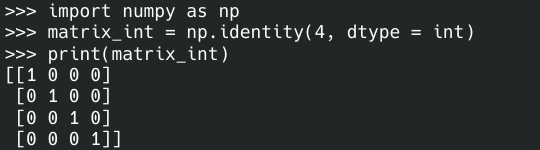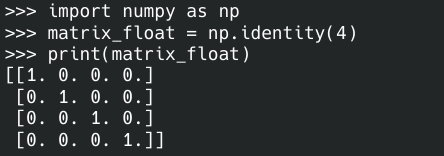# Create Identity Matrix With Python

Yahya Irmak Apr 12, 2022

In linear algebra, the n-dimensional identity matrix is an n × n square matrix with ones on the major diagonal and zeros everywhere else. This article will explain how to create an identity matrix with the `NumPy` library of the Python programming language.

## Create Identity Matrix With Python

`NumPy` is a Python programming language library to create large, multidimensional arrays and matrices. Install the `NumPy` library with the `pip3 install numpy` command to create the identity matrix.

This library’s `identity()` function takes a number as an argument and returns an identity array with that number of rows and columns. You can optionally specify the data type of the output. It uses `float` by default.

A 4x4 identity matrix with the `integer` data type is created in the example below.

``````import numpy as np

matrix_int = np.identity(4, dtype = int)
print(matrix_int)
``````If you do not specify the `dtype` parameter, the matrix is created with the `float` data type.

``````import numpy as np

matrix_float = np.identity(4)
print(matrix_float)
``````Author: Yahya Irmak

Yahya Irmak has experience in full stack technologies such as Java, Spring Boot, JavaScript, CSS, HTML.